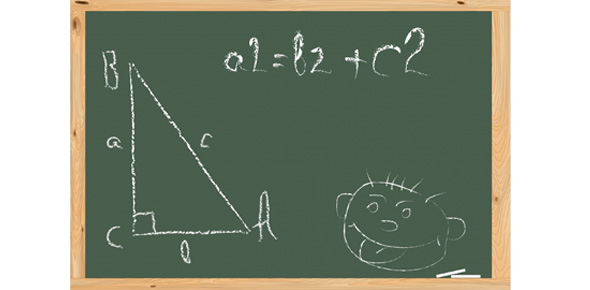# Review: Circumference And Pythagorean Theorem

Approved & Edited by ProProfs Editorial Team
The editorial team at ProProfs Quizzes consists of a select group of subject experts, trivia writers, and quiz masters who have authored over 10,000 quizzes taken by more than 100 million users. This team includes our in-house seasoned quiz moderators and subject matter experts. Our editorial experts, spread across the world, are rigorously trained using our comprehensive guidelines to ensure that you receive the highest quality quizzes.
| By Phillipssg
P
Phillipssg
Community Contributor
Quizzes Created: 13 | Total Attempts: 6,312
Questions: 5 | Attempts: 100Settings• 1.

### What is the circumference of a circle with a radius of 3 feet? Round to the nearest whole number.

• A.

19 feet

• B.

9 feet

• C.

15 feet.

A. 19 feet
Explanation
Circumference = pi*D
D= 2*r
D = 2*3 = 6 feet
C = 3.14*6 = 18.84 = 19 feet.

Rate this question:

• 2.

### What formula is used to solve for the sides of a right triangle?

• A.

Circumference

• B.

Pythagorean theorem

• C.

Area

B. Pythagorean theorem
Explanation
The Pythagorean theorem is used to solve for the sides of a right triangle. It states that in a right triangle, the square of the length of the hypotenuse (the side opposite the right angle) is equal to the sum of the squares of the lengths of the other two sides. This theorem is a fundamental concept in geometry and is widely used to find missing side lengths in right triangles.

Rate this question:

• 3.

### What are the three sides of a triangle called when we are calculating a pipe offset?

• A.

• B.

45, 45, 90

• C.

Travel, run, set

C. Travel, run, set
Explanation
When calculating a pipe offset, the three sides of a triangle are referred to as travel, run, and set. The travel represents the horizontal distance, the run represents the vertical distance, and the set represents the diagonal distance or the hypotenuse of the triangle. These terms are commonly used in pipefitting and plumbing to determine the correct measurements and angles for creating pipe offsets.

Rate this question:

• 4.

### If the set of a pipe is 12 cm and the run of the pipe is 15 cm, what is the travel of the pipe? Round your answer to the nearest whole number.

• A.

27 cm

• B.

19 cm

• C.

15 cm

B. 19 cm
Explanation
The travel of the pipe refers to the total distance covered by the pipe. In this case, the set of the pipe is 12 cm and the run of the pipe is 15 cm. The travel of the pipe can be calculated by adding the set and run together. Therefore, the travel of the pipe is 12 cm + 15 cm = 27 cm. However, the question asks to round the answer to the nearest whole number, so the correct answer is 19 cm.

Rate this question:

• 5.

### What is defined as the distance around a circle?

• A.

Area

• B.

Perimeter

• C.

Circumference

C. Circumference
Explanation
The distance around a circle is defined as its circumference. The circumference is the total length of the boundary or the outer edge of the circle. It can be calculated using the formula 2πr, where r is the radius of the circle. The term "perimeter" is more commonly used for polygons, while "area" refers to the space enclosed within the boundary of a shape. Therefore, the correct answer is circumference.

Rate this question:

Related TopicsBack to top